#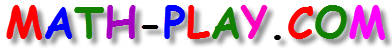The 1st grade math games on our website provide students and teachers with an exciting way of practicing basic math facts. The math problems are aligned to the Common Core Standards. Drills alone are boring, although students need a lot of practice to become more proficient in math. First grade students love to play; why not let them have fun and learn something new every day?

When playing these games, 1st grade students will practice one of the following math skills:

• Understand place value.
• Solve problems involving addition and subtraction.
• Add and subtract within 20.
• Work with addition and subtraction equations.
• Understand and apply properties of operations and the relationship between addition andsubtraction.
• Tell and write time.
• Represent and interpret data.
• Reason with shapes and their attributes.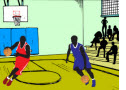Counting to Ten Basketball Game

In this interactive basketball game, 1st grade students will practice counting to ten.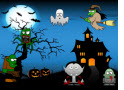Sums to 10 Halloween Math Game Try this exciting math game and practice your skills. For each correct answer, you will enter a bonus round where you can earn points by smashing monsters.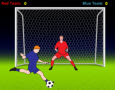Addition and Subtraction Soccer Game1st Grade Halloween Math Game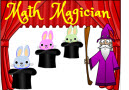Math Magician Subtraction Game
The subtraction problems in this exciting Math Magician Subtraction Game provide students plenty of practice subtracting numbers to 20.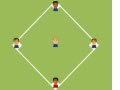Baseball Math Addition Game
Children can have lots of fun as they solve the addition problems in this exciting Baseball Math Addition Game.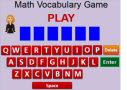1st Grade Vocabulary Game

In this interactive and fun online game, 1st grade students will practice important math vocabulary words and definitions.Math Magician Addition Game
Kids will be provided with loads of fun when they solve the addition problems in this exciting Math Magician Addition Game.Baseball Math Place Value Game
Make a game of learning place value by playing this exciting Baseball Math Place Value Game.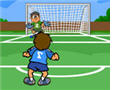Addition Soccer Game

In this online soccer game, 1st grade students will practice adding one-digit numbers.

Return from the Classroom Math Games page to Math Play homepage.